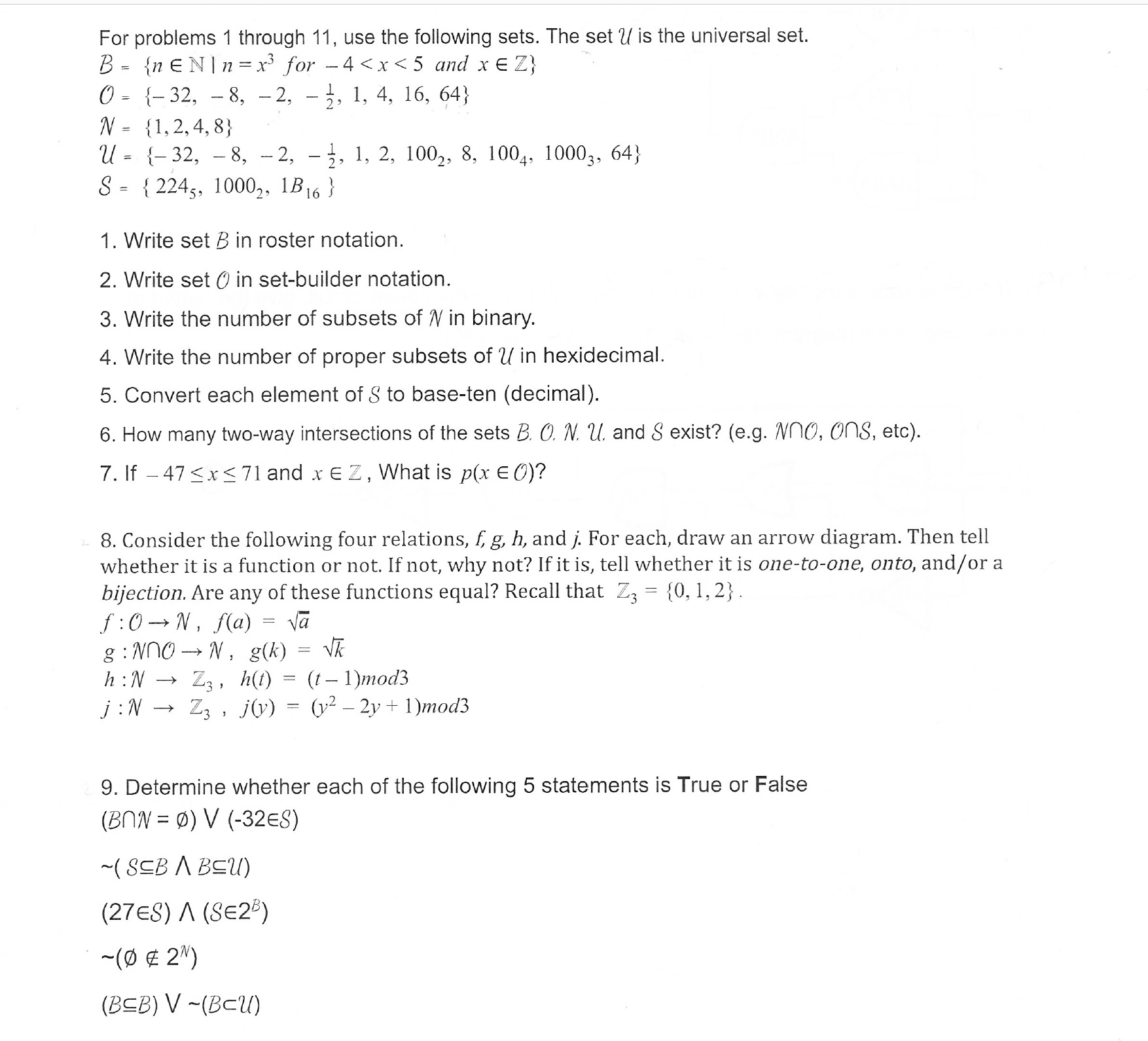# For problems 1 through 11, use the following sets. The set U is the universal set.0- -32, - 8, -2, - 3, 1, 4, 16, 64)N-1,2,4,8)U= {-32,-8,-2, .. 1, 2, 100-, 8. 1004. 10003, 64)S-2245, 1000,, B16)1. Write set B in roster notation.2. Write set O in set-builder notation3. Write the number of subsets of N in binary4. Write the number of proper subsets of U in hexidecimal.5. Convert each element of S to base-ten (decimal)6. How many two-way intersections of the sets B. O. N u. and S exist? (e.g. Nno, ons, etc)7. If -47

Question
93 views

I need the explanation for number 6 and 7help_outlineImage TranscriptioncloseFor problems 1 through 11, use the following sets. The set U is the universal set. 0- -32, - 8, -2, - 3, 1, 4, 16, 64) N-1,2,4,8) U= {-32,-8,-2, .. 1, 2, 100-, 8. 1004. 10003, 64) S-2245, 1000,, B16) 1. Write set B in roster notation. 2. Write set O in set-builder notation 3. Write the number of subsets of N in binary 4. Write the number of proper subsets of U in hexidecimal. 5. Convert each element of S to base-ten (decimal) 6. How many two-way intersections of the sets B. O. N u. and S exist? (e.g. Nno, ons, etc) 7. If -47
check_circle

Step 1
1. The sets are given as B, O, N, U, S in which U is the Universal set.

In order to find the number of two-way intersections like (N∩B, O∩U, etc.) of the sets B, O, N, U, S, obtain the permutations as first place has 5 choices and second place will have 4 choices.

Step 2

The number of two-way intersection of the sets B, O, N, U, S is 10. However, out of 10, only 6 intersections are possible that are U∩B, U∩O, U∩N, N∩B, N∩O, and O∩B.

Step 3

Set S has no intersection with any other set as the elements are the numbers with some bases that do not even matches wit...

### Want to see the full answer?

See Solution

#### Want to see this answer and more?

Solutions are written by subject experts who are available 24/7. Questions are typically answered within 1 hour.*

See Solution
*Response times may vary by subject and question.
Tagged in